## Friday, 8 August 2014

### CHAPTER 15-Worked Out Examples 5

 Example: 13
 In a quadrilateral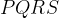$PQRS$,$\overrightarrow {PQ} = \vec a,\,\overrightarrow {QR} = \vec b$ and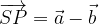$\overrightarrow {SP} = \vec a - \vec b$. If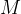$M$ is the mid-point of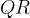$QR$ and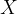$X$ is a point on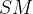$SM$ such that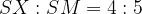$SX : SM = 4 : 5$, prove that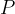$P$,$X$ and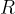$R$ collinear.
 Solution: 13
Since no position vectors have been specified in the question (only the sides have been specified), there is no loss of generality in assuming that$P$ is the origin$\vec 0$.We have,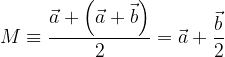$M \equiv \dfrac{{\vec a + \left( {\vec a + \vec b} \right)}}{2} = \vec a + \dfrac{{\vec b}}{2}$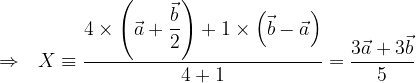$\Rightarrow \,\,\,\, X \equiv \dfrac{{4 \times \left( {\vec a + \dfrac{{\vec b}}{2}} \right) + 1 \times \left( {\vec b - \vec a} \right)}}{{4 + 1}} = \dfrac{{3\vec a + 3\vec b}}{5}$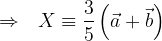$\Rightarrow \,\,\,\, X \equiv \dfrac{3}{5}\left( {\vec a + \vec b} \right)$
Thus,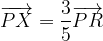$\overrightarrow {PX} = \dfrac{3}{5}\overrightarrow {PR}$
implying that$P$$X$ and$R$ are collinear.
 Example: 14
It is known that in a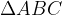$\Delta ABC$ with centroid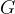$G$, circumcentre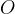$O$ and orthocentre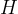$H$,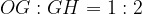$OG:GH = 1:2$
Let$P$ be any point in the plane of$\Delta ABC$. Prove the following assertions:
 (a)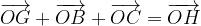$\overrightarrow {OG} + \overrightarrow {OB} + \overrightarrow {OC} = \overrightarrow {OH}$ (b)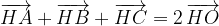$\overrightarrow {HA} + \overrightarrow {HB} + \overrightarrow {HC} = 2\,\overrightarrow {HO}$ (c)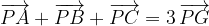$\overrightarrow {PA} + \overrightarrow {PB} + \overrightarrow {PC} = 3\,\overrightarrow {PG}$
 Solution: 14-(a)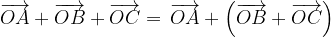$\overrightarrow {OA} + \overrightarrow {OB} + \overrightarrow {OC} = \,\overrightarrow {OA} + \left( {\overrightarrow {OB} + \overrightarrow {OC} } \right)$$= \,\overrightarrow {OA} + 2\overrightarrow {OD}$ (since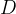$D$ is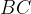$BC$‘s mid-point)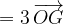$= 3\,\overrightarrow {OG}$ (Since$G$ lies on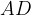$AD$ and divides it in the ratio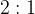$2:1$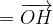$= \overrightarrow {OH}$ (Since$O$,$G$ and$H$ are collinear and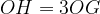$OH=3OG$
 Solution: 14-(b)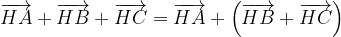$\overrightarrow {HA} + \overrightarrow {HB} + \overrightarrow {HC} = \overrightarrow {HA} + \left( {\overrightarrow {HB} + \overrightarrow {HC} } \right)$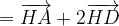$= \overrightarrow {HA} + 2\overrightarrow {HD}$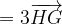$= 3\overrightarrow {HG}$ (Same logic as above)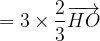$= 3 \times \dfrac{2}{3}\overrightarrow {HO}$ (again, same as above)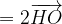$= 2\overrightarrow {HO}$
 Solution: 14-(c)
For any arbitrary point$P$ in the plane of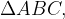$\Delta ABC,$ we have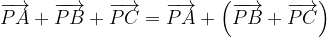$\overrightarrow {PA} + \overrightarrow {PB} + \overrightarrow {PC} = \overrightarrow {PA} + \left( {\overrightarrow {PB} + \overrightarrow {PC} } \right)$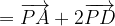$= \overrightarrow {PA} + 2\overrightarrow {PD}$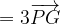$= 3\overrightarrow {PG}$
Go over the solution again if you find any part of it confusing.